# A cylindrical tank

A cylindrical tank can hold 44 cubic meters of water. If the radius of the tank is 3.5 meters, how high is the tank?

h =  1.1433 m

### Step-by-step explanation: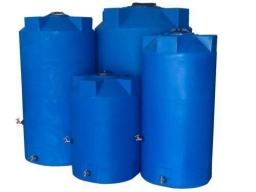Did you find an error or inaccuracy? Feel free to write us. Thank you!

Showing 1 comment:Elle
It was very hard but i will tryTips to related online calculators
Tip: Our volume units converter will help you with the conversion of volume units.

## Related math problems and questions:

• Cylindrical tank9.6 hl of water is poured into a cylindrical tank with a bottom diameter of 1.2 m. What height in centimeters does the water reach?
• Cylindrical tankCylindrical tank holds 600hl water and is deep 2.5 m. Calculate the diameter of the cylinder.
• Mike built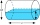Mike built a cylindrical water tank with a radius of about 1.8 m and height of 2 meters. How many liters of water will it hold?
• Gasoline tank cylindrical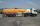What is the inner diameter of the tank, which is 8 m long and contains 40 cubic meters of gasoline?
• Cylinder containerThe cylindrical container with a diameter of 1.8 m contains 2,000 liters of water. How high does the water reach?
• Hectoliters of waterThere are 942 hectoliters of water in a cylindrical tank with an inner diameter of 6 m. The water reaches two thirds of the depth of the tank. Calculate its depth.
• WaterInto a full cylindrical tank high 3 m with a base radius of 2.5 m, we insert cuboid with dimensions 1.7 m, 1.3 m, 1.9 m. How many liters of water will overflow out?
• The solar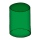The solar heating tank has the shape of a rotating cylinder. When the tank is in a horizontal position, the water in the tank wets 4/5 each tank bases. If we place the tank in a vertical position, the water in the tank will reach up to 1.2 m. Calculate th
• Tank diameterA cylindrical tank has a volume of 60 hectoliters and is 2.5 meters deep. Calculate the tank diameter.
• Cylindrical tank 2If a cylindrical tank with volume is used 12320cm raised to the power of 3 and base 28cm is used to store water. How many liters of water can it hold?
• Water tankWater tank shape of cuboid has dimensions of the bottom 7.5 meters and 3 meters. How high will reach the water in the tank will flow 10 liters of water per second and the inflow will be open 5/6 hour? (Calculate to one decimal place and the results give i
• The tankThe tank is full up to 4/5 of the total height and contains 240 hl of water. The area of the base is 6 square meters. What is the height of the tank?
• Tank and waterWe poured a cylindrical tank with 3.5 liters of water. If the tank base diameter is 3 dm, how height is the water level in?
• Water tank300hl of water was filled into the tank 12 m long and 6 m wide. How high does it reach?
• Tank B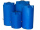Tank B has the same volume as Tank A. The volume of one section of Tank B is 4545 cubic feet. What is the volume, in cubic feet, of the other section of Tank B? Total volume of both tanks A+B is 15000 ft3. Enter your answer in the box.
• Horizontal Cylindrical SegmentHow much fuel is in the horizontal cylindrical segment tank with a length of 10m, the width of level 1 meter, and the level is 0.2 meters below the tank's upper side?
• LiquidHow much liquid does the rotary cylinder tank hold with base diameter 1.2m and height 1.5m?